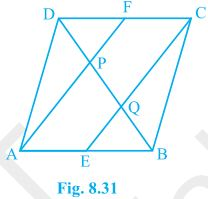# In a parallelogram ABCD, E and F are the mid-points of sides AB and CD respectively. Show that the line segments AF and EC trisect the diagonal BD.Given: ABCD is a parallelogram. E and F are the mid-points of sides AB and CD respectively.

To prove: AF and EC trisect the diagonal BD

ABCD is a parallelogram

AB || CD

AE || FC

AB = CD (Opposite sides of parallelogram ABCD)

⇒½ AB = ½ CD

⇒ AE = FC (E and F are midpoints of side AB and CD)

AECF is a parallelogram (AE and CF are parallel and equal to each other)

AF || EC (Opposite sides of a parallelogram)

From ΔDQC, F is mid point of side DC and FP || CQ (as AF || EC).

P is the mid-point of DQ (Converse of mid-point theorem)

⇒ DP = PQ — (i)

Similarly,

From ΔAPB,

E is midpoint of side AB and EQ || AP (as AF || EC).

Q is the mid-point of PB (Converse of mid-point theorem)

⇒ PQ = QB — (ii)

From equations (i) and (ii),

DP = PQ = BQ

Therefore, the line segments AF and EC trisect the diagonal BD.

Hence proved(0)(0)